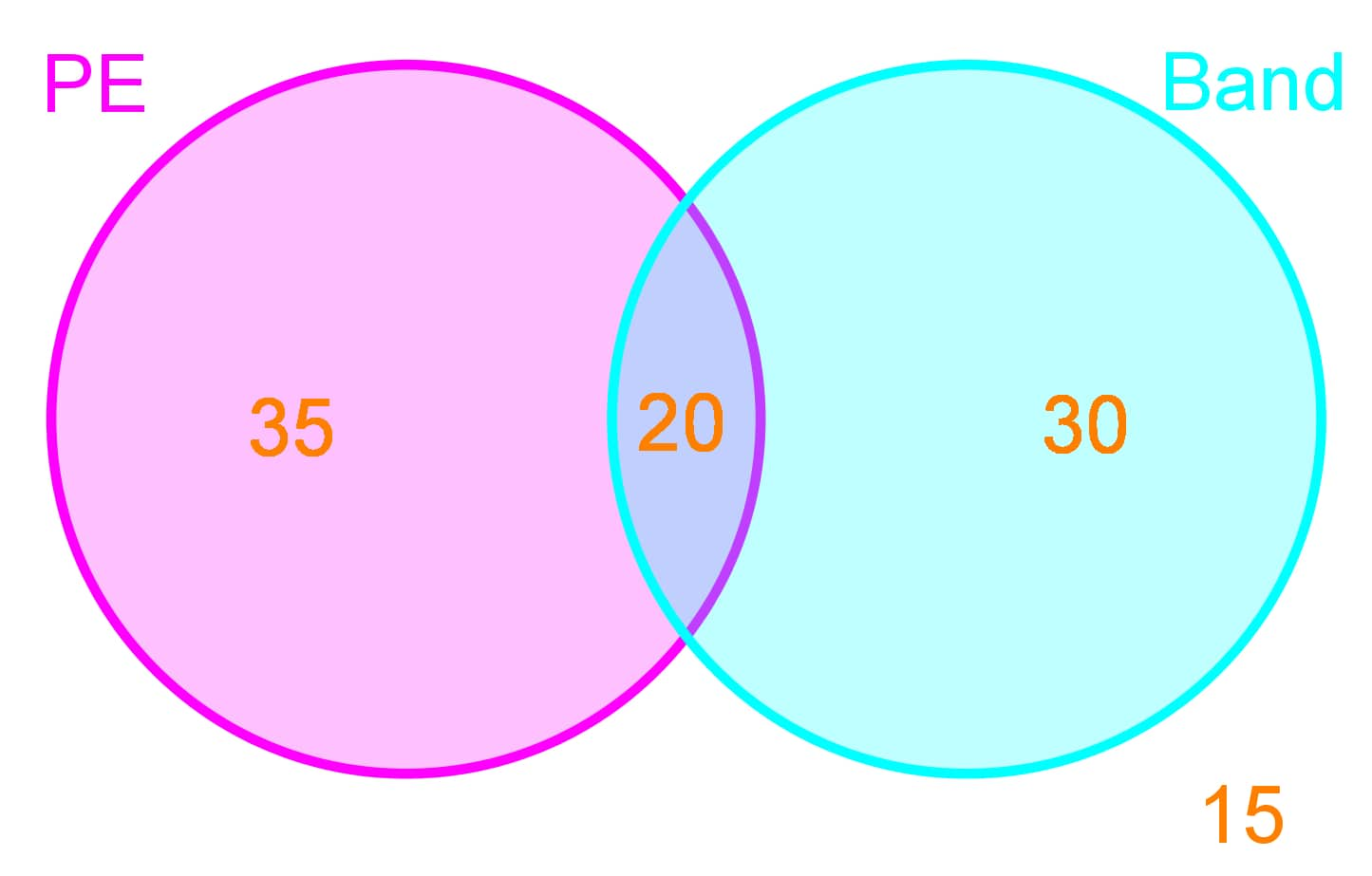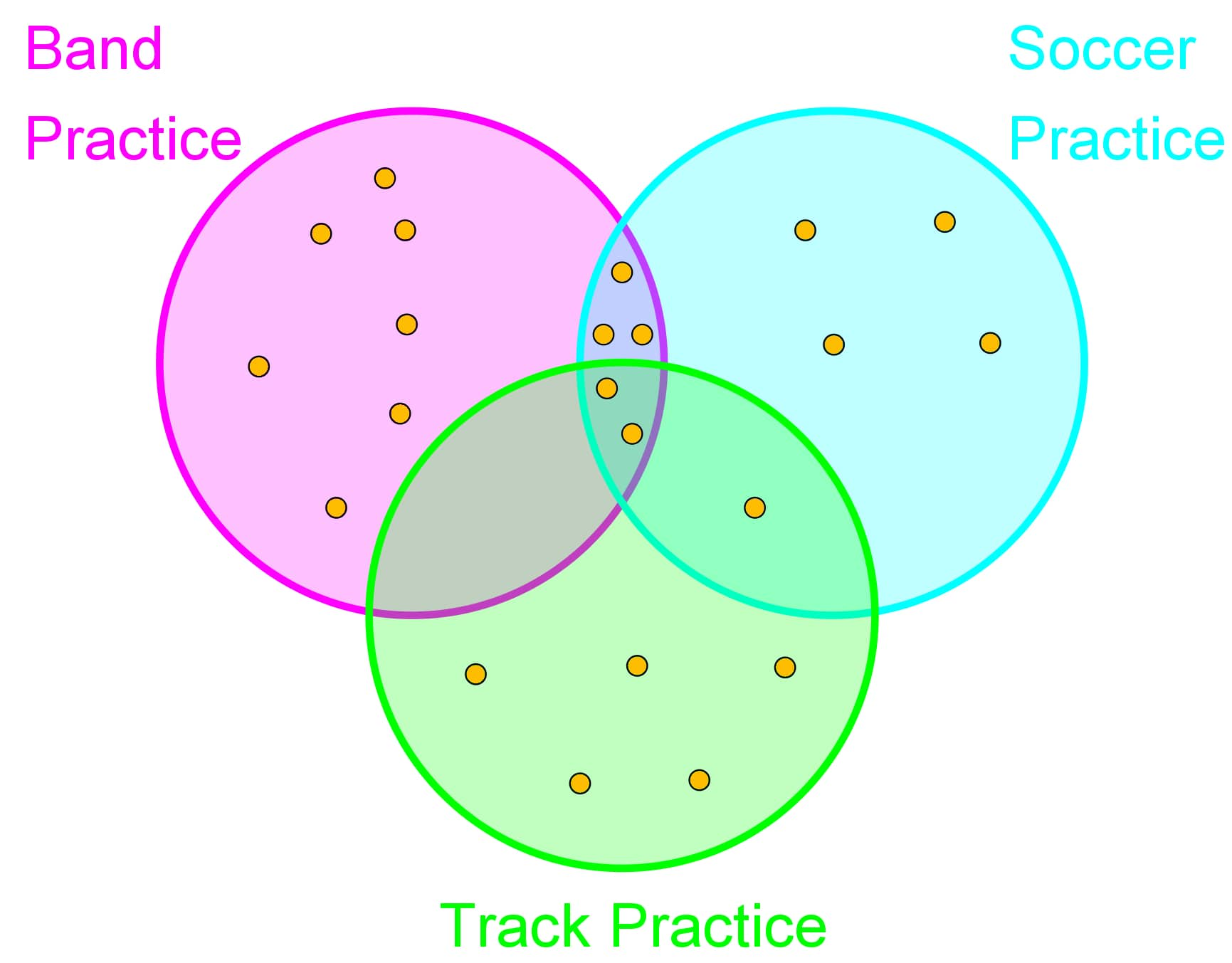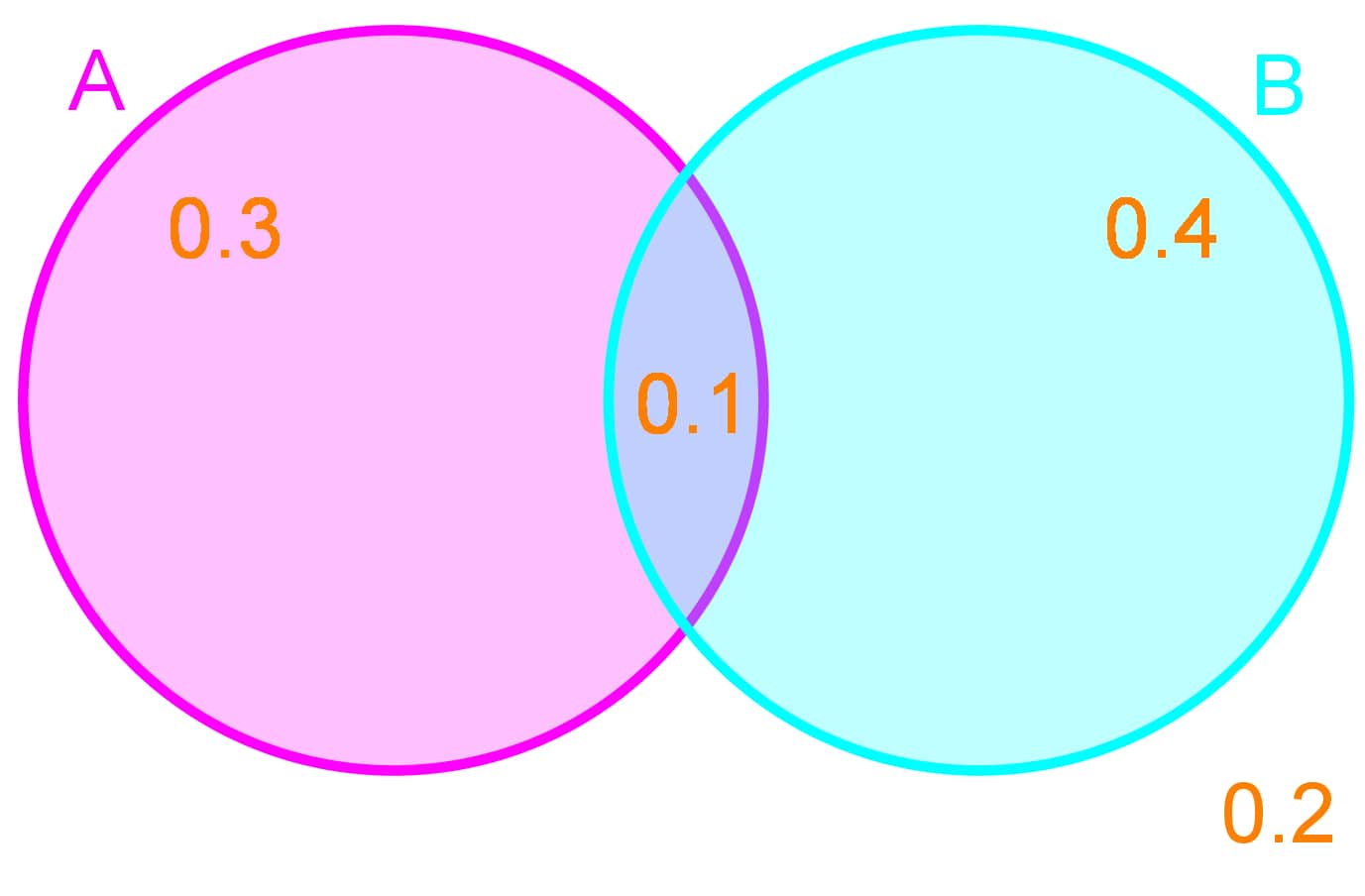# Probability with Venn diagrams

### Probability with Venn diagrams

#### Lessons

Venn Diagrams can be a useful tool to represent and solve probability questions

Sample space:
All possible outcomes of an experiment

Union($\cup$, "or"):
A$\cup$B is the event that either A occurs or B occurs or they both occur

Intersection ($\cap$, "and"):
A$\cap$B is the event that both A occurs and B occurs

Addition Rule:
A shortcut formula to finding is: A$\cup$B = A + B – A$\cap$B

Complement $(A^c)$:
All outcomes EXCEPT the event $P(A^c )=1-P(A)$
• Introduction
How do we calculate probability with Venn Diagrams?

• 1.
Venn Diagrams Represent Events
Out of a school of 100 students the number of students enrolled in PE and Band class is given below:So there are 35 students who are taking only PE classes, 30 students who are taking only Band classes, and 20 students who are taking both PE and Band classes. There are also 15 students who are not enrolled in PE or Band classes.
a)
Replicate this Venn diagram in terms of probabilities of picking a random student from any of these classes.

b)
What is the probability of picking a student who is in PE class?

• 2.
Union and Intersection with Venn Diagrams
The following dots represent students who attend each after school activity:a)
How many students attend only Band Practice, what is the probability of picking a student who attends only Band Practice?

b)
How many students attend Soccer Practice AND Band Practice, what is the probability of picking these students?

c)
How many students attend Soccer Practice AND Band Practice AND Track Practice, what is the probability of picking these students?

d)
How many students attend Track Practice OR Soccer Practice, what is the probability of picking these students?

e)
How many students attend Band Practice OR Soccer Practice, but not Track Practice? What is the probability of picking these students?

• 3.
Addition Rule
From a deck of cards 10 cards are drawn at random. From these 10 cards the probability of picking a king is 0.3 and the probability of picking a heart is 0.5. The probability of picking a card that is both a king and a heart (the king of hearts) is 0.1.
a)
Represent these probabilities as a Venn Diagram

b)
What is the probability of picking a king that isn't a heart?

c)
What is the probability of picking a heart that isn't a king?

d)
What is the probability of picking a king OR a heart?

• 4.
Determining the Complement of Events
The probabilities of certain events are given in the Venn Diagram below:a)
What is the probability of A occurring?

b)
What is the probability of A NOT occurring?

c)
What is the probability of A occurring or B occurring?

d)
What is the probability of neither A nor B occurring?